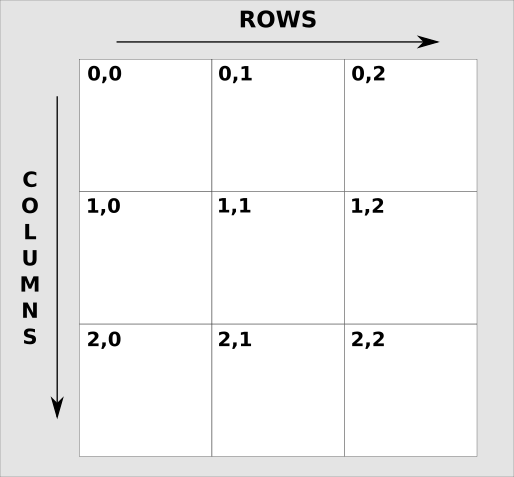# Turn Rows Into Columns With The Ruby Transpose Method

Today you are going to learn how to deal with grids in Ruby using the Ruby transpose method.

Imagine that you have a perfect grid, let’s say a 3×3 square, in the form of a multi-dimensional array.

And you want to take the rows & convert them into columns.

Why would you want to do that?

One use is for the classic game: tic-tac-toe.

You store your board as a grid. Then to find a winning move you have to check the rows, columns & diagonals.

The problem is that if you are storing your grid as an array you only get direct access to the rows.

## Columns The Hard Way

By “direct access” I mean that you can go over your array (with `each`, `map`, etc.) without having to use more array indexing than necessary.

Let’s see an example!

Here’s a grid:

```grid = [
[1,2,3],
[4,5,6],
[7,8,9]
]
```

Here’s a visual I made for you:You could get the columns by referencing the indexes.

For example, the first column would be:

```[grid, grid, grid]

# [1, 4, 7]
```

But the first row is just this:

```grid

# [1, 2, 3]
```

How can we make working with columns as easy as working with rows?

## Columns The Easy Way

The easy way to do this is by using the Array#transpose method.

Example:

```columns = grid.transpose
```

Yes, that’s all you have to do!

Now you can get the first column like this:

```columns

# [1, 4, 7]
```

As you can see knowing a lot of methods can save you a lot of work 🙂

## Tic-Tac-Toe

I’m not going to explain the whole thing. I just want to show you how this method can apply to a real project.

To win a game of tic-tac-toe you need to fill a row, a column or a diagonal.

Here’s the code for checking rows:

```def check_rows
@board.each { |row| return row.first if all_equal?(row) }
end
```

And here’s is the code for columns:

```def check_columns
@board.transpose.each { |row| return row.first if all_equal?(row) }
end
```

Notice how the only difference is the `transpose` method!

Here’s the `all_equal?` method:

```def all_equal?(row)
return if row.first == nil

row.each_cons(2).all? { |x,y| x == y }
end
```